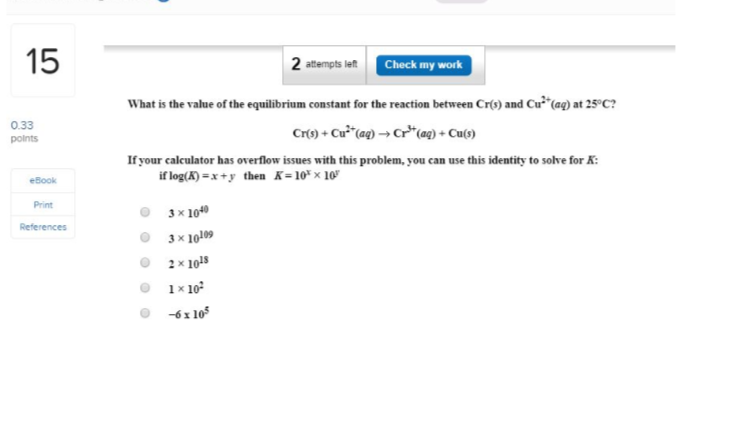# What is the value of the equilibrium constant for the reaction between Cr(s) and (aq) at 25-C7 Cr(s)+Cu2(aq)----Cr3(aq)+Cu(g) If your calculator has overflow issues with this problem, can use this identity to solve for K: If log(k)=x+y then k=10 X 10